# Volume + time - math problems

#### Number of problems found: 49

• Volume per timeHow long does fill take for a pump with a volume flow of 200 l per minute fill a cube-shaped tank up to 75% of its height if the length of the cube edge is 4 m?
• Tributaries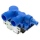The pool can be filled with two different tributaries. The first inflow would fill the pool in 18 hours, both in 6 hours. How many hours would the pool filled with a second inflow?
• A filterIt is a pool with a volume of 3500 liters. The filter filters at 4m cubic per hour. How many minutes would it filter the entire pool?
• Blueberries collecting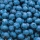Marie collects blueberries 2 l in 1 hour. Began collecting at 8.00 pm, Joe collects 1.5 l in 1 hour. Peter 1 l for 1 hr. And they began to gather at 08.30 pm. Together they collect 10 l. At what time will they all have collected?
• Two water containers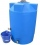In the first container, there are 200 m3 of water and in the second 40 m3. The first container will flow down at a rate of 10 m3 water per hour. At the same time flows to the second rate of 5 m3 per hour. After how many hours there will be three times les
• Three pumps together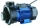One pump fills the tank in 1.5 hours, the second in 2 hours, and the third in 3 hours 20 minutes. How many minutes will the tank fill with three pumps if they work simultaneously?
• Three tributaries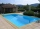It is possible to fill the pool with three tributaries. The first would take 12 hours, the second 15 hours, and the third 20 hours. The day before the summer season began, the manager opened all three tributaries simultaneously. How long did it take to fi
• Pump680 liters of water were pumped in 8 minutes. How many liters was spent in 56 minutes?
• Five inlets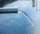The tank can be filled with five equally powerful inlets. If the tank is filled by four of these inlets, it takes a total of 30 minutes to fill one-third of the tank. How many minutes does it take to fill an empty tank if it is filled with all five inlets
• Two pipesHow long will the pool be filled with a double supply pipe if it takes the pool to fill the first pipe by 4 hours longer and the second pipe 9 hours longer than both pipes open at the same time?
• Three pumpsWe are filling the pool. The first pump would be filled in 12 hours, the second pump in 15 hours. If all three pumps were running at the same time, it would fill the pool for 4 hours. How long would the pool fill only with the third pump?
• Orlík hydroelectric plantThe Orlík hydroelectric power plant, built in 1954-1961, consists of four Kaplan turbines. For each of them, the water with a flow rate of Q = 150 m3/s is supplied with a flow rate of h = 70.5 m at full power. a) What is the total installed power of the p
• Inlet and outletThe pool has a capacity of 50 hl. The inlet pipe flows in 1 minute 1.25 hl, and the waste pipe outlet is draining the full pool in 50 minutes. How long will take the empty the full pool when both the inlet and outlet are opened at the same time?
• Water levelHow high is the water in the swimming pool with dimensions of 37m in length and 15m in width, if an inlet valve is opened for 10 hours flowing 12 liters of water per second?
• Scientific notation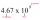Approximately 7.5x105 gallons of water flow over a waterfall each second. There are 8.6x104 seconds in 1 day. Select the approximate number of gallons of water that flow over the waterfall in 1 day.
• Water tankIn an empty fire tank, 2150 hl of water jetted in 5 hours. How many hectoliters of water was jetted every hour? How many hectoliters of water was in the tank after three hours?
• Water flowHow much water flow in pipe with a diameter of 16 cm in 1 hour if the water velocity is 2.5 m/s?
• Oil tank and pipes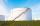The underground oil tank can be filled by two oil pipelines. The first is filled in 72 hours and the second in 48 hours. How many hours from the moment when first pipeline began to fill the oil is it necessary to start filling it with the second to fill i
• Basic formExpressed the ratios of values in the basic form: 0,5 t : 1,2 kg 200 l : 0,15 m3 12 t : 3600 kg 500 kg : 2,5 t 0,9 kg : 500 g 3,6 m : 240 cm 1200 mm : 2,4 m 300 l : 0,3 m3 6 min 30 s : 900 s
• Fuel mileage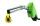You have 3/8 tank of fuel. Estimate fuel consumption @ 6mpg. Estimate speed @ 50mph. Your fuel tank holds 200 gals. Figure out fuel mileage.

Do you have an interesting mathematical word problem that you can't solve it? Submit a math problem, and we can try to solve it.

We will send a solution to your e-mail address. Solved examples are also published here. Please enter the e-mail correctly and check whether you don't have a full mailbox.

Please do not submit problems from current active competitions such as Mathematical Olympiad, correspondence seminars etc...

Tip: Our volume units converter will help you with the conversion of volume units. Do you want to convert time units like minutes to seconds?